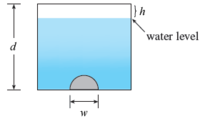Hydrostatic Force Problem (Calc 2)

quinn

New member
I have been really stuck on this concept from my calculus course. If anyone's familiar with these types of problems and can post a solution that would be great.

Ex) A vertical dam has a semicircular gate as shown in the figure. The total depth d of the figure is 36 m, the height h of air above the water level is 6 m, and the width w of the gate is 10 m. Find the hydrostatic force against the gate. (Round your answer to the nearest whole number. Use 9.8 m/s^2 for the acceleration due to gravity. Recall that the weight density of water is 1000 kg/m^3.)MarkFL

Super Moderator
Staff member
To begin, what is the relationship between pressure and force?

quinn

New member
Pressure is equal to the force over a certain area.

•MarkFL

MarkFL

Super Moderator
Staff member
Pressure is equal to the force over a certain area.
Yes, pressure $$P$$ is defined to be force $$F$$ per area $$A$$:

$$\displaystyle P=\frac{F}{A}$$

And this implies then:

$$\displaystyle F=AP$$

So, then how is pressure at some point beneath the surface of some uncompressible fluid related to it density $$\rho$$ and its depth $$x$$?

•Otis

quinn

New member
Is it the integral of (ρ ⋅ density ⋅ g ⋅ x)dx over 0≤x≤36?

MarkFL

Super Moderator
Staff member
Is it the integral of (ρ ⋅ density ⋅ g ⋅ x)dx over 0≤x≤36?
You're jumping way ahead, but no. I'm trying to take you from basic definitions and concepts to develop the solution.

Pressure$$P$$ is a function of depth $$x$$, given a constant mass density $$\rho$$:

$$\displaystyle P(x)=\rho gx$$

And so we may now write:

$$\displaystyle dF=\rho gx\;dA$$

Now, because pressure varies as the depth, we may take horizontal strips along the surface of the gate as $$dA$$:

$$\displaystyle dA=\ell\,dx$$

where $$\ell$$ is the length of a strip. We need this length as a function of the depth $$x$$. Using the circular geometry of the gate, can you determine this?

quinn

New member
ℓ=1/2(2πrx); w=10m so r=5m
ℓ=1/2(2·5πx)
ℓ=5πx
Would this be the correct function of dA? x being the depth: x=36ft

Last edited:

MarkFL

Super Moderator
Staff member
The depth at the top of the gate (in terms of the given variables) is:

$$\displaystyle x_1=d-h-\frac{w}{2}$$

And the depth at the bottom of the gate is:

$$\displaystyle x_2=d-h$$

Now, we know:

$$\displaystyle \ell\left(x_1\right)=0$$

$$\displaystyle \ell\left(x_2\right)=w$$

But, we need a function that expresses $$\ell$$ for all $$x_1\le x\le x_2$$. Imagine $$x$$ as a vertical axis and $$\ell$$ as a horizontal axis with the origin at the surface of the fluid directly above the center of the base of the gate. Then the boundary or perimeter of the gate will be the top half of a circle of radius $$\displaystyle \frac{w}{2}$$ centered at $$\left(0,d-h\right)$$. The full circle has the equation:

$$\displaystyle \ell^2+(x-(d-h))^2=\frac{w^2}{4}$$

Hence:

$$\displaystyle \ell(x)=2\sqrt{\frac{w^2}{4}-(x-(d-h))^2}=\sqrt{w^2-(2(x-(d-h)))^2}$$

Does this make sense to you so far?

quinn

New member
Yes, so now we come to integrate (ρ ⋅ density ⋅ g ⋅ ℓ)dx over 0≤x≤36 correct?

MarkFL

Super Moderator
Staff member
Yes, so now we come to integrate (ρ ⋅ density ⋅ g ⋅ ℓ)dx over 0≤x≤36 correct?
No, what we have now is:

$$\displaystyle dF=\rho gx\sqrt{w^2-(2(x-(d-h)))^2}\,dx$$ where $$x_1\le x\le x_2$$.

Hence:

$$\displaystyle F=\rho g\int_{x_1}^{x_2}x\sqrt{w^2-(2(x-(d-h)))^2}\,dx$$

or:

$$\displaystyle F=\rho g\int_{d-h-\frac{w}{2}}^{d-h}x\sqrt{w^2-(2(x-(d-h)))^2}\,dx$$

At this point, I would consider the substitution:

$$\displaystyle u=x-(d-h)\implies du=dx$$

And, our definite integral becomes:

$$\displaystyle F=\rho g\int_{-\frac{w}{2}}^{0}(u+d-h)\sqrt{w^2-(2u)^2}\,du$$

Can you proceed?

quinn

New member
Thank you for the explanation.